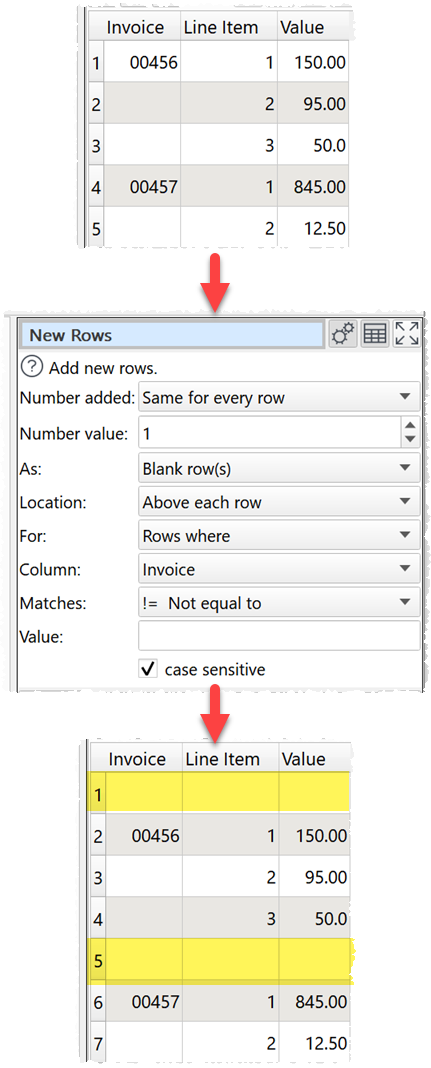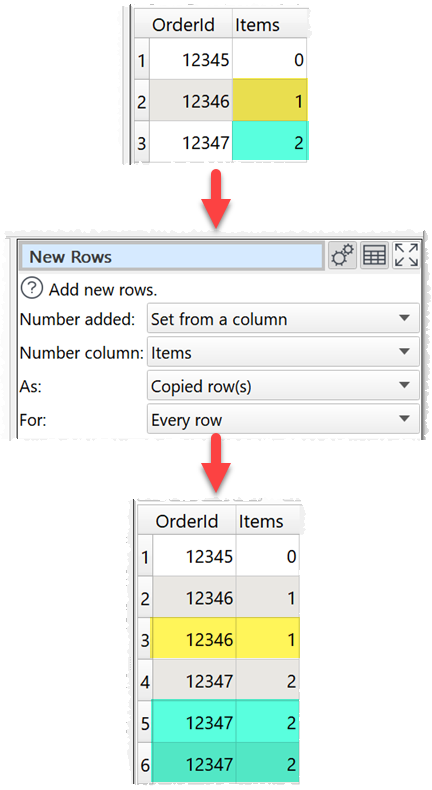﻿ Reference > Transforms > New Rows

# New Rows

## Examples

Add a blank row above every row with an invoice number:Copy each row the number of times in the 'Items' column:One.

## Options

Set Number added according how many rows to add for each existing row.

oSet Number value to the number of new rows to create for each existing row (available when Number added is Same for every row).

oSet Number column to get the number of new rows to create for each existing row from a column (available when Number added is Set from a column).

Set As to add either blank rows or copies of existing rows.

Set Location depending on whether you want new rows added above or below existing rows (available when As is Blank row(s)).

Set For depending on whether you wish to add rows to all rows or only rows that meet certain conditions.

## Notes

You can use Calculate or Javascript transforms to calculate the number for the Number column. E.g. to subtract 1 from the final number of copies wanted to give the number of new rows.